## Saturday, January 26, 2013

### Review: MyScript Calculator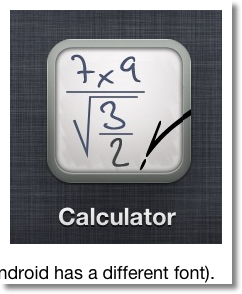MyScript Calculator Review

Available for: iOS, Android
Company: Vision Objects
Cost: Free
Latest Update: 12/19/2012 (iOS), 1/24/2013 (Android)

The program lets you literally write calculations with your finger or stylus. Results are calculated automatically. Up to three decimal places are shown for approximations. Expressions, which include fractions and square roots, are built dynamically. You can even insert characters or draw "scratches" to remove characters.

It has a learning curve, because the app has to recognize what you write. A suggestion is to go slow at first, and let the app recognize each character. Patience is needed. However, I now can fulfill my fantasy to write calculations and have them execute.

Order of operations are similar to graphing calculators. (Negation is low on the order of operations)

I don't think describing what this app does using words, does it justice. I definitely encourage you to download it and try it for yourself.

Both the iOS and Android version (Android as of today) have the following features:

* Arithmetic and Exponents
* Percent, and it works properly! (36 - 10% = 32.4)
* Square Root, which the surd expands to accommodate long expressions under the root
* Support for the constants π, e, and ϕ (the golden ratio)
* Logarithmic functions (log to any base, ln, e^x)
* Trigonometric functions
* Absolute value
* Parenthesis
* Ability to solve equations by use of the ? and = symbols
* Factorial
* Tweet, email, or post results on Facebook (and probably other social networks)

I am excited about the future of these type of this app.

What I like to see in future updates is:
* Ability to show answers beyond 3 decimals** (see below)
* Numerical calculus: derivatives and integrals
* Matrices and complex number support, I can see the potential

Rating: 5 stars out of 5

I'll leave you with some screenshots of the MyScript app.

** Alexander Oestert of HP Forum (HP Museum of Calculators) pointed out that in the Android version that you can have decimal settings up to 6 places. (1/27/2013)

Until next time,

Eddie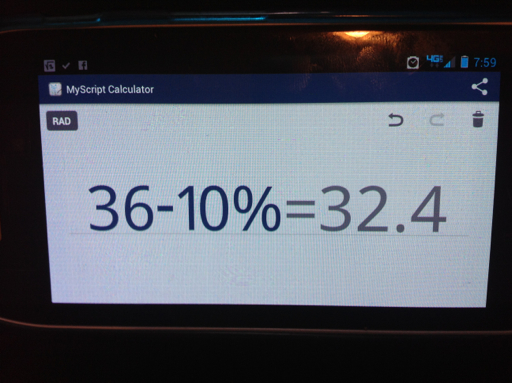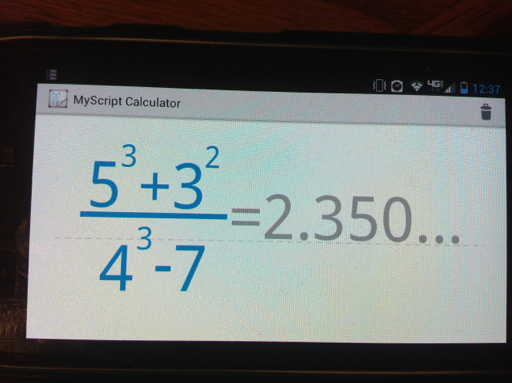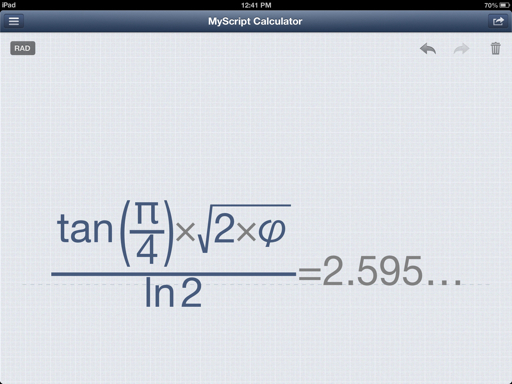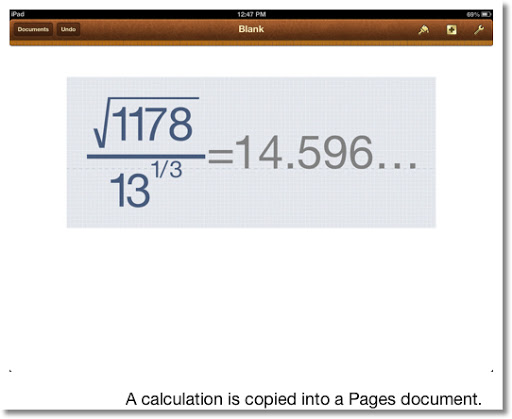This blog is property of Edward Shore. 2013

### Speed Test - Casio CalculatorsHi everyone! Today I am blogging from home.

Last time I posted a speed test for various Texas Instruments, which you can go to by clicking on this link.

For each calculator, I timed how long does the calculator take until it "counts down" from 2013 to 0. I ran the program on each calculator twice.

The Program TEST2013

TEST2013:
2013 → A
Lbl 1
A - 1 → A
A > 0 ⇒ Goto 1 : 1

The program returns 1 when completed.

For the Casio ClassPad 330, I had to modify the program a bit, as the ⇒ symbol means "store" (instead of "jump") for the ClassPad.

2013 ⇒ a
Lbl one
a
a - 1 ⇒ a
If a > 0
Then
Goto one
IfEnd

Results

I will start from the oldest made model. Thanks to rskey.org and Wikipedia for the dates.

fx-7000GA (1990): 31.11 sec, 30.89 sec
(The fx-7000GA is as close to the original 7000G as I will probably get).

fx-6300G (1991): 38.91 sec, 38.90 sec

CFX-9850G (1997): 28.58 sec, 28.00 sec

fx-9750G Plus (2002): 28.50 sec, 28.44 sec

fx-3650P (2002) Solar Powered: 3 min 6 sec, 3 min 6.31 sec

ClassPad 330, Operating System 3.04.4000 (2007): 35.89 sec, 36.01 sec

Prizm (fx-CG 10) (2010), Operating System 1.04.3200 : 9.53 sec, 9.44 sec
(I am very happy with this!)

Note that the fx-3650P is a solar powered-calcualtor. I had no idea that this model was released in 2002. I had to get it from eBay, as it is not sold in stores in the United States.

I did not test any of the modern fx-9860g family (GII, Slim, etc...), but I guess that these calculators are fast of the Prizm. From these results, it is apparent that Casio knows how to maximize their processor speed.

Next time I will review the calculator app MyScript. Until next time,

Eddie

This blog is property of Edward Shore. 2013

## Thursday, January 24, 2013

### TI-8x Speed Test - A slowdown for the TI-84+?

Here is a speed test between newer and older Texas Instruments calculators. Please keep in mind this is one way to compare speeds. I ran the program on each calculator listed twice. The test is to see how fast the calculator "counted" to 2013.

Appropriately, the test program is named TEST2013.

TEST2013:*

0 →A
ClrHome
Lbl 1
Output(1,1,A)
IS>(A,2013)
Goto 1

*The TI-81 does not have an Output command, so Disp A was used instead.

TI-81: 52.70 sec, 52.36 sec

TI-82: 38.73 sec, 38.78 sec

TI-83+ Silver Edition, Operating System 1.14:
17.92 sec, 17.96 sec

TI-85: 47.91 sec, 47.43 sec

TI-84+, Operating System 2.55MP, MathPrint Mode On:
1 min 20.03 sec, 1 min 19.91 sec
(yes, this is shocking)

TI-84+ Silver Edition running on a TI nSpire via TI-84+ Keypad, Operating System 2.54MP, MathPrint On:
46.93 sec, 46.15 sec
(a second faster than the TI-85)

I don't know why the tests ran slower on the 84+s. At first, guessed that it is that the MP 2 is more complex than MP 1 and requires a great deal of RAM to operate. If I recall correctly, almost all the TI-8x series run on a Zilog Z80 chip.

A post written in 2010 from ticalc.org stated that there have been reports that programs run slower on the TI-84+ with operating system 2.53MP (or greater). Kevin Ouellet responded that the slow down issue is resolved if MathPrint is turned off.

Source: TI-OS 2.53 Released for the TI-84+ Family, 2/15/2010, written by Nikky. Retrieved 1/23/2013.

Let's put that to the test. If you don't know, on the TI-84+, you can turn MathPrint on and off by pressing the MODE key, scroll up or down to the second page. Choose CLASSIC. In classic mode, the F1, F2, and F4 shortcut menus are still available, except all input is linear.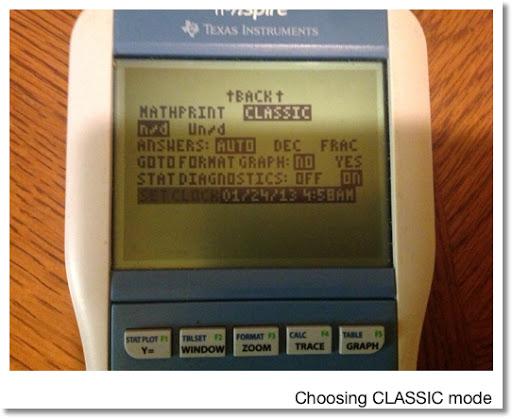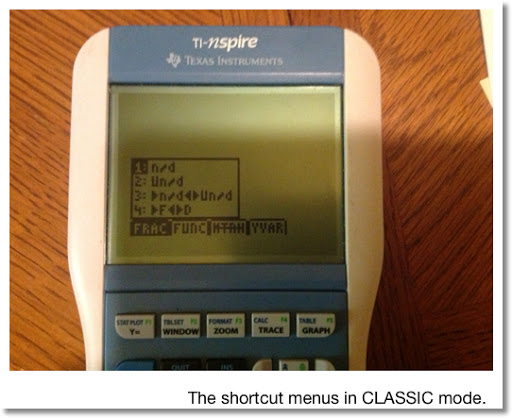Running these tests yields these results:

TI-84+, Operating System 2.55MP, MathPrint Off (CLASSIC):
27.02 sec, 27.00 sec

TI-84+ Silver Edition running on a TI nSpire via TI-84+ Keypad, Operating System 2.54MP, MathPrint Off (CLASSIC):
31.03 sec, 31.00 sec

A significant difference for the TI-84+. To compare: 1 minute 20 seconds with MathPrint On, only 27 seconds with it off.

The motto of the story is, if you have a TI-84+ and want to run a program, consider turning MathPrint off, and set it in CLASSIC mode instead.

I am curious to see if TI addressed this issue with the new TI-84+ C Silver Edition coming out this Spring.

Next time, I will post speed times for a handful of Casio calculators.

This blog is property of Edward Shore. 2013

## Sunday, January 20, 2013

### Converting Polar Equations to Parametric Equations

Good Sunday!

This blog will show how to transform polar equations, in the form of r(θ) to a pair of parametric equations, x(t) and y(t).

Tools We Need

x = r * cos θ
y = r * sin θ

Let θ = t.

For each example, we will change each polar equation and display a graph for each form. The polar form, colored blue, is on top; the parametric form, in red, is on the bottom. (A TI nSpire-CX is used for the pictures)

Example (I):

r = 2*θ

Then θ = r/2 and let θ = t.

x = r * cos t
x = 2 * t * cos(r/2)
x = 2 * t * cos((2*t)/2)
x = 2 * t * cos t

Similarly,

y = r * sin t
y = 2 * t * sin(r/2)
y = 2 * t * sin t

To summarize:
r = 2*θ

is equivalent to

x = 2 * t * cos t
y = 2 * t * sin t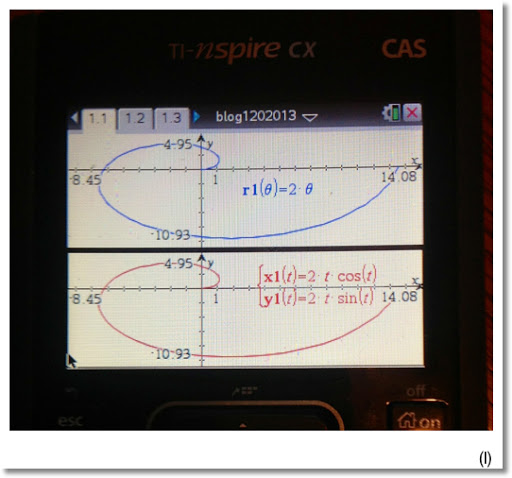Example (II):

r = e^(2 * θ)

Then θ = 1/2 * ln r and let θ = t.

And...

x = r * cos t
x = e^(2*t) * cos(1/2 * ln r)
x = e^(2*t) * cos(1/2 * ln(e^(2*t)))
x = e^(2*t) * cos(1/2 * 2 * t)
x = e^(2*t) * cos t

y = r * sin t
y = e^(2*t) * sin(1/2 * ln r)
y = e^(2*t) * sin(1/2 * ln(e^(2*t)))
y = e^(2*t) * sin t

To summarize:
r = e^(2*θ)

is equivalent to:

x = e^(2*t) * cos t
y = e^(2*t) * sin t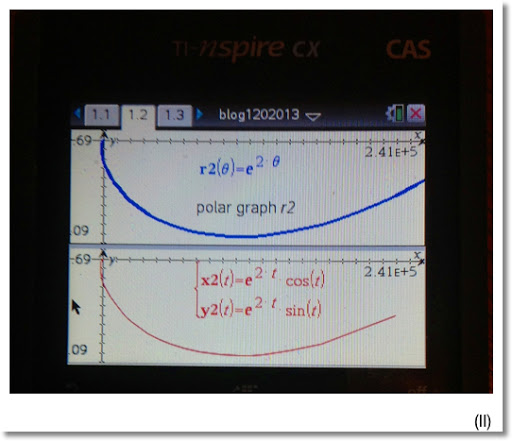Example (III):

r = 2 cos θ

Then:

θ = arccos(r/2) = cos⁻¹(r/2)

Then, with θ=t...

x = r * cos t
x = 2 * cos t * cos(cos⁻¹(r/2))
x = 2 * cos t * cos(cos⁻¹(2*cos(t)/2))
x = 2 * cos t * cos t
x = 2 * cos² t

And... (some trigonometric identities are required)

y = r * sin t
y = 2 * cos t * sin(cos⁻¹(r/2))

Note: sin(cos⁻¹ x) = √(1 - x²)

y = 2 * cos t * √((1 - (r/2)²)
y = 2 * cos t * √(1 - r²/4)
y = 2 * cos t * √(1 - (4 cos² t)/4)
y = 2 * cos t * √(1 - cos² t)

Note: sin² x + cos² x = 1

y = 2 * cos t * sin t

Note: sin(2*x) = 2 * cos x * sin x

y = sin (2*t)

To Summarize:
r = 2 cos θ

is equivalent to:

x = 2 * cos² t
y = sin (2*t)Until next time, take care!

Eddie

This blog is property of Edward Shore. 2013

## Wednesday, January 16, 2013

### Length of a Polynomial Segment - Part 1; and the HP 50g Program MPFIT

The next two blog entries are going to deal with finding the length of a polynomial segment.

Polynomial Segments

What is a polynomial segment? I could not find a formal definition, so I'll describe it is this: similar to a line segment where a pair of points are connected with a line, a polynomial segment is a set of points that are connected by polynomial curve. The degree of the polynomial is determined by the number of points, less one. For instance, if we have 3 points, the polynomial that connects them the order 2 (quadratic). A cubic polynomial (order 3) is used to connect a set of 4 points.

The Goal

We have a set of points {(x0, y0), (x1, y1), (x2, y2),... (xn, yn)}. Connect the points using a polynomial. What is the length of that segment?

General Method

1. Find the polynomial to connect the set of points. Order of the elements is important. This is determined by using the matrix calculation (X^T X)^-1 (X^T y) to determine the coefficients. The resulting matrix contains the coefficients of the polynomial; with each term being a coefficient of x of increasing power, from 0 to n-1.

2. Use the arc length formula ∫ ( √ (1 + (f'(x))^2 ) dx, a, b) where a is the minimum of the x values and b is the maximum of the x values.

We recommend using a calculator or math software that can handle matrices and integrals for this procedure.

But what is X and y?

y represents a vector of y-values of the set of points.

X is a Vandermonde Matrix. A Vandermonde matrix is a n × n matrix is formed with each row containing a geometric progression of each term. The first column, each term is raised to the 0th power, the second column each term to the 1st, the third column each term is raised to the second power, and so on until the nth row where each term is raised to the n-1 power.

For instance, a Vandermonde matrix consisting of the set [a, b, c, d] would look like this: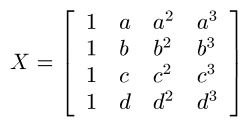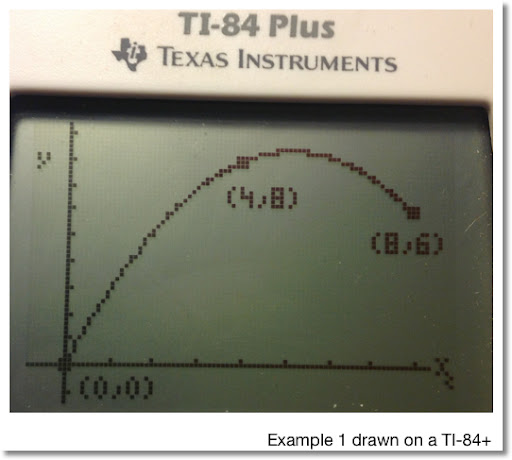Example 1

Find a polynomial segment that fits the points (0,0), (4,8), and (8,6). Find the length of that segment. (See the diagram above).

The x values are [0, 4, 8] with xmin = 0 and xmax = 8. The y values are [0, 8, 6]. Let X be the Vandermonde matrix with the set [0, 4, 8] and the vector y be represented by the corresponding y-values.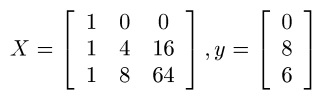Now we need to calculate (X^T X)^-1 (X^T y).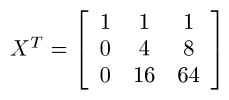Then: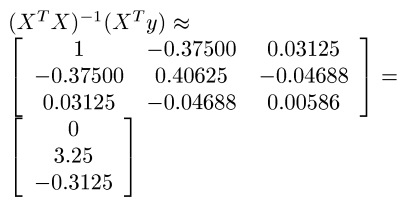The resulting matrix contains the coefficients of the required polynomial. Since the vector has 3 entries, the order of the polynomial is 3-1=2.

Going top-down:
0 is the constant (coefficient of x^0),
3.25 is the coefficient of x,
and -0.3125 is the coefficient of x^2.

Our polynomial is f(x) = 3.25x - 0.03125x^2.

To find the length, use the arc length formula using end points xmin = 0 and xmax = 8. We find that the length of the polynomial segment connecting (0,0), (4,8), and (8,6) is about 14.23920.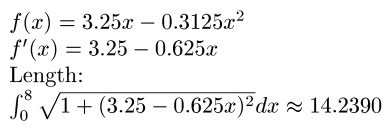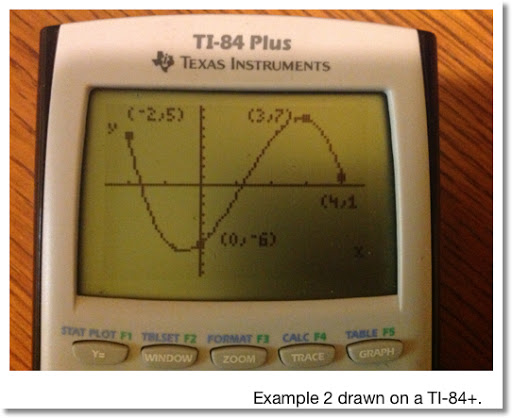Example 2

What is the length of the polynomial segment connected with the points (-2,5), (0,-6), (3,2), and (4,1). We are working with 4 points, the required polynomial has the order 4-1=3. (Cubic polynomial)

The Vandermonde matrix X with the vector y are as follows: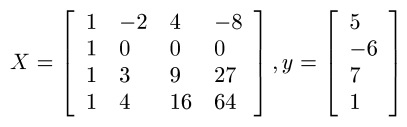Calculating (X^T X)^-1 (X^T y) yields (approximately):

[ [ -6 ]
[ 2.98333 ]
[ 2.725 ]
[ -0.75833 ] ]

To form the cubic polynomial:
-6 is the constant,
2.98333 is the coefficient of x,
2.725 is the coefficient of x^2, and
-0.75833 is the coefficient of x^3.

With xmin = -2 and xmax = 4, we find the length of this segment is approximately 32.60952. (See below).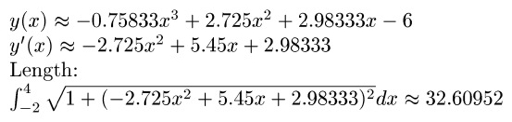HP50g Program MPFIT

The HP 50g program MPFIT fits a polynomial to the set of points {(x0, y0), (x1, y1), (x2, y2), ... , (x_(n-1), y_(n-1))}.

Input:
2: vector of x coordinates
1: vector of y coordinates

Output:
2: Coefficients in matrix form [ [a0] [a1] [a2] ... [a_(n-1)] ]
1: Polynomial of X in the form a0 + a1 * X + a2 * X^2 + ... + a_(n-1) * X^(n-1)

Program MPFIT (size 183.5 bytes)

<< DUP SIZE OBJ→ DROP → MX MY N
<< 'X' PURGE
MY OBJ→ 1 + →ARRY
MX VANDERMONDE
LSQ → MR
<< MR DUP
{1, 1} GET
2 N FOR K
MR K 1 2 →LIST GET
'X' K 1 - ^ * +
NEXT >> >> >>

Example:
Input:
2: [1, 3, 6]
1: [6, 3, 5]

Output:
2: [[8.8] [-3.23333333333] [0.43333333333]]
1: 8.8+-3.23333333333*X+0.43333333333*X^2

Which means the polynomial fit for the given points is (approximately):

8.8 - 3.23333x + 0.43333x^2

(Screen shots of this example are shown below)

To get the length of the segment with the HP 50g, first note the xmin and xmax values, which are 1 and 6, respectively. This keystroke (in RPN Mode) should do the trick:

[ ' ] [ X ] [ENTER]
[RS] [COS] ( δ )
[LS] [ √ ] (x²)
1 [ + ] [ √ ]
1 [ENTER]
6 [ENTER]
3 [LS] [EVAL] (PRG) [F1] (STACK) [NXT] [F1] (ROLL)
[ ' ] [ X ] [ENTER]
[RS] [TAN] ( ∫ )

Length = 7.75662832829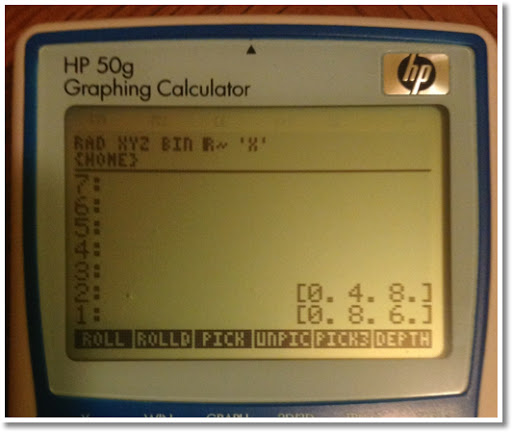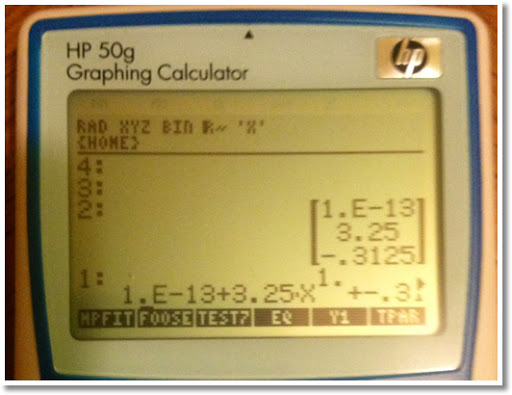My next blog entry will contain programs for the TI-84+ and Casio Prizm (fx series) calculators.

Have a great day everyone,

Eddie

This blog is property of Edward Shore. 2013

### Length of a Polynomial Segment - Part 2 (TI-84+/Casio); From Coffee Klatch in San DimasToday I am coming to you from Coffee Klatch in San Dimas, CA. This is one of my most favorite places to hang out, and have great coffee and food.

Today's blog is the second part of the polynomial segment blog. Here is a link to Part 1.

To Recall:

A polynomial segment is a set of points that is connected by a polynomial.

To find a perfectly fit polynomial to fit n points:

1. Let X be the Vandermonde matrix of order (n-1) × (n-1) for the following: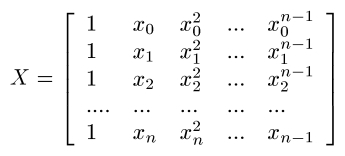2. Let y be the vector of y values.
3. The coefficient matrix is calculated as (X^T X)^-1 (X^T y). The result is a vector of coefficients of x^k where k = 0 to n-1.

On most graphing (and some scientific calculators), you can accomplish this task in two ways:

1. Use matrix operations. This is good for any size polynomial.
2. Take advantage of the curve fitting functions LinReg (to fit 2 points), QuadReg (to fit 3 points), CubicReg (to fit 4 points), and QuartReg (to fit 5 points). *Regression models and names vary for each calculator.

Once the polynomial, f(x) is found, find the arc length is found by: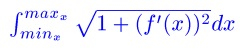The limits of the integral are the minimum and maximum x values, respectively.

Here are two programs that accomplish the task of finding a polynomial fit for 3, 4, or 5 points for the TI-84+ (and its family), and the Casio Prizm (and its fx-9xxx family).

TI-84+

POLYARC
1/12/2013
Fit a polynomial to a set of three, four, or five points.
Find the arc length of the polynomial from the end points.

Example 1: (0,0), (4,8), (8,6)
Results: y = -0.3125x^2 + 3.25
Length = 14.23920

Example 2: (0,3), (2,-1), (4,2), (6,0)
Results: y = -0.25x^3 + 2.375x^2 - 5.75x + 3
Length = 12.46834

Example 3: (0,0), (1,1), (2,0), (3,-1), (4,0.5)
Results: y ≈ -0.02083333x^4 + 0.20833333x^3 - 1.77083333x^2 + 2.54166666x
Length = 6.36142413

Program POLYARC (327 bytes)
Input "NO OF PTS(3-5)", N
If N<3 or N>5
1/0
N → dim(L1)
N → dim(L2)
For(K,1,N)
Disp K
Input "X=", X
Input "Y=", Y
X → L1(K)
Y → L2(K)
End
If N=3
Then
fnInt(√(1+(2aX+b)²),X,min(L1),max(L1)) → L
Pause {a, b, c}
End
If N=4
Then
CubicReg L1, L2
Pause {a, b, c, d}
fnInt(√(1+(3aX² + 2bX + c)²), X, min(L1), max(L1)) → L
End
If N=5
Then
QuartReg L1, L2
Pause {a, b, c, d, e}
fnInt(√(1 + (4aX³ + 3bX² + 2cX + d)² , X, min(L1), max(L2)) → L
End
Disp "LENGTH="
Pause L

Where to find:
a, b, c, d, e: VARS, 5 (Statistics), right, right, [2, 3, 4, 5, and 6 respectively]
You need the lower case a, b, c, d, and e!

Note: No "bending backwards" is allowed

Casio Prizm

POLYARC
1/12/2013
Fit a polynomial to a set of three or four points.
Find the arc length of the polynomial from the end points.

Example 1: (0,0), (4,8), (8,6)
Results: y = -0.3125x^2 + 3.25
Length = 14.23920

Example 2: (0,3), (2,-1), (4,2), (6,0)
Results: y = -0.25x^3 + 2.375x^2 - 5.75x + 3
Length = 12.46834

Example 3: (0,0), (1,1), (2,0), (3,-1), (4,0.5)
Results: y ≈ -0.02083333x^4 + 0.20833333x^3 - 1.77083333x^2 + 2.54166666x
Length = 6.36142413

Program POLYARC (352 bytes):
"NO. OF POINTS(3,4,5)"? → N
N<3 Or N>5 ⇒ 1 ÷ 0
N → Dim List 1
N → Dim List 2
For 1 → K To N
K ◢
"X"? → List 1[K]
"Y"? → List 2[K]
Next
If N = 3
Then QuadReg List 1, List 2
∫( √(1 + (2aX + b)² ), Min(List 1), Max(List 1)) → L
{a, b, c} ◢
EndIf
If N=4
Then CubicReg List 1, List 2
∫( √(1 + (3aX ² + 2bX + c)² ), Min(List 1), Max(List 1)) → L
{a, b, c, d} ◢
EndIf
If N=5
Then QuartReg List 1, List 2
∫ ( √(1 + (4aX^3 + 3bX ² + 2cX + d)² ), Min(List 1), Max(List 2)) → L
{a, b, c, d, e} ◢
EndIf
"LENGTH="
L

How to Access:
a, b, c, d, and e: VARS, STAT (F3), GRAPH (F3), F1 (F2, F3, F4, and F5 respectively)

Note: No "bending backwards" allowed

Enjoy the day and I'll talk to you soon!

Eddie

This blog is property of Edward Shore. 2013

## Saturday, January 12, 2013

### Swörk Coffee and My Feelings About the Upcoming TI-84+ C and Casio ClassPad fx-CP400

Hi everyone! Today I coming to you from Swörk Urban Coffee Bar in Eagle Rock, CA (Los Angeles). It is a beautiful and sunny - but cold - Saturday morning.in 2013, Casio and Texas Instruments are going to offer updates two of their graphing calculators: Casio's ClassPad and TI-84+.

Just in case you are not familiar with Casio ClassPad's series - in the United States, they are not sold in office stores and popular shopping marts (they have to bought online), here is a short introduction:

In 2003, Casio released the ClassPad 300 and a few years later, the 330, which I have. The ClassPad is significantly larger than the typical graphing calculator. The calculator has a 4.8" grayscale screen and a super basic keyboard. Almost everything that is done using the calculator's soft-keyboard and menu. The keyboard is accessible by touch or by the provided stylus.

The features of the 330 are rich (partial list):
* Over 500,000 bytes of RAM (that's a lot of programs!) and 5.4 MB ROM
* A mini-USB port
* Screen Resolution: 160 × 240
* CAS (Computer Algebra System)
* Geometry Application
* 3D and Differential Equation Graphing
* The ability to select equations, drag them, drop them, copy them, and graph them instantly.

In preparation for this blog entry, I dusted my ClassPad 330 and got reacquainted with it. To be honest, I forgot how awesome the ClassPad is. Here are some pics of the 330: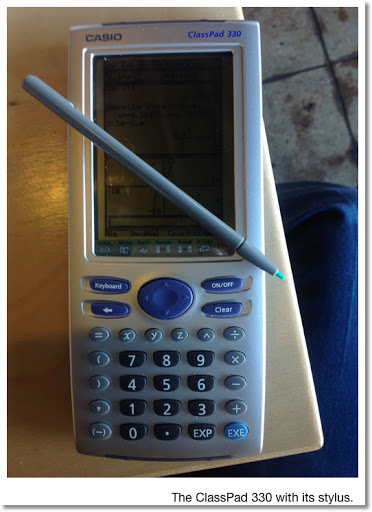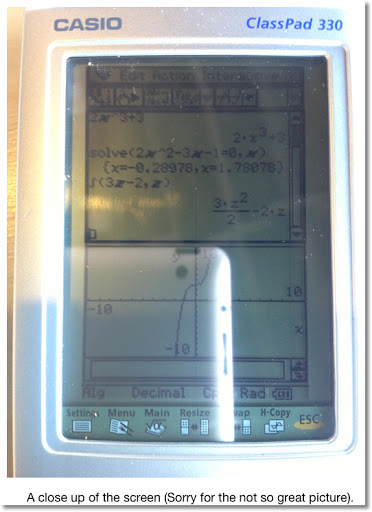The ClassPad fx-CP400 is featured to have:
* A true-touch screen - which must mean it is more touch friendly. A stylus will still be provided.
* The calculator can operate in portrait or landscape mode.
* The screen will be in color, which I presume to be similar to the current Casio Prizm.
* Resolution increases to 328 × 528.
* The soft keyboard will have bigger keys.
* 500 KB RAM, 5.5 MB ROM, 24 MB USB Flash Drive - same programming space as of the 330.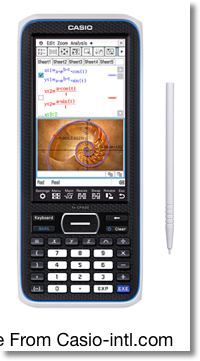I am excited to get the fx-CP400 when it gets released. This looks like it will be a solid calculator and become the high-end Casio calculator. I am curious to about how the ClassPad operates in landscape mode. 99% of the time I use my iPad in landscape mode and enjoy the interface much better than the portrait landscape.

Cost? I read anywhere from \$150 to \$200, the former most likely.

News release of the fx-cp400 (11/27/2012)

TI-84+ Color

During Spring 2013, Texas Instruments will release an update to the TI-84+ series. The big update is that the new TI-84+ will have a color screen, similar to the current TI nSpire CX line.In the case that you are not familiar with the TI-84+, the current model looks like what is displayed in the picture, only with a grayscale screen and a bigger font.

Some of the features of the upcoming TI-84+ C Silver Edition are:
* Memory: The ROM increases to 3.5 MB, but the RAM decreases to 21,000 bytes. This means less space for programming than the current TI-84+.
* Screen Resolution increases from 96 × 24 to 320 × 240. The resolution will be the same as the TI nSpire CX (and CX CAS).
* The TI-84+ C Silver Edition will have a rechargeable battery. TI states that a charge can last from five days to two weeks - depending on use.
* While the TI-84+C Silver Edition has an updated operating system, the commands and functionality will be similar to the TI-80s family.
* The calculator's modes will be displayed on top of the screen at all times.

I am not happy about the decrease in programming memory! 24,000 bytes is towards the low end already. The TI-84+ is really not for writing long programs - short quick ones will do. The backlit screen is a nice plus. Two reasons why I like backlit screens: (1) the screen is easier to read and (2) I get better pictures for the blog.

The TI-84+ will continue to be an application based calculator. I am hoping for in the update is a grid-like icon menu when I press the APPS key instead of a list. I am happy that the TI-84+ C Silver Edition will have the modes listed on top of the screen, as well as a battery indicator - both welcome additions.

Cost? I am read that prices are speculated between \$100 to \$150. I am really hoping its more towards \$100, since TI's nSpire CX has a \$150 price tag. I would guess about \$130. Still - if TI releases the TI-84+ C in March - that would most likely become my birthday present.

Texas Instrument's Press Release: TI-84+ C Silver Edition

Article from Tech Powered Math regarding the TI-84+ C Silver Edition

Verdict

At this point I am more excited about the fx-CP400 than the TI-84+ C Silver Edition. Most likely I plan to buy both calculators. I am happy that newer calculators have color, backlit screens. Not only they give the calculator a 21st century look, they are easier to read.

That is it for now, take care and have a great day!

Eddie

This blog is property of Edward Shore. 2013

## Wednesday, January 9, 2013

### Bringing Circles Together

Question: Start with two circles with radii of 2.64 (of whatever units of length you like) each.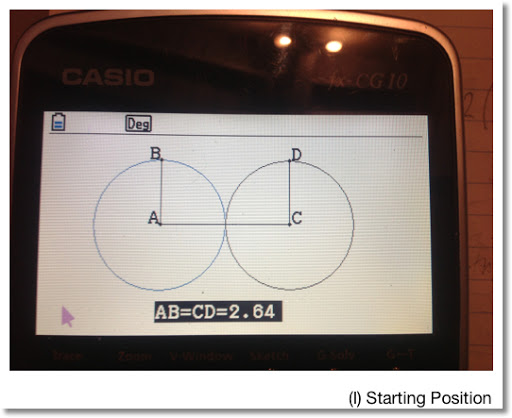Move the circle outlined in blue (on the left) to the right. How is the total area of the figure affected?

Formulas We Need:

Area of a Circle: A = π * r^2

Area of an Ellipse: A = π * a * b
Where a = length of the semi-major axis
And b = length of the semi-minor axis

The circles in (I) are joined together, each with a radius of 2.64. Calculating the total area is fairly easy:

A = 2 * π * 2.64^2 = 43.79128832 ≈ 43.79

Keep this result in mind.

Note that I will refer to each picture on this blog entry by (I), (II), (III), etc.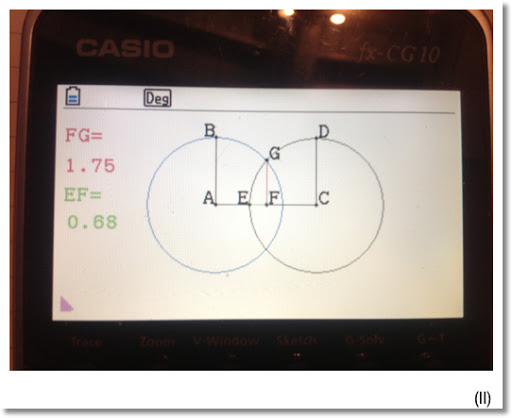Using the Geometry Mode of the Casio Prizm, I drew two circles shown in (I). Moving the blue circle to the right 10 "clicks" and taking measurements yield two overlapping circles. The total area is still the area of the circles less the overlap of the two circles. The overlap is in the form of an ellipse.

Note that line segments EF and FG represent the semi-axis of the overlap ellipse.

The measure of the segments EF and FG, according to the Prizm geometry mode, were 0.68 and 1.75 respectively.

The area of the resulting figure to (II):

A = 2 * π * 2.64^2 - π * 1.75 * 0.68 ≈ 40.05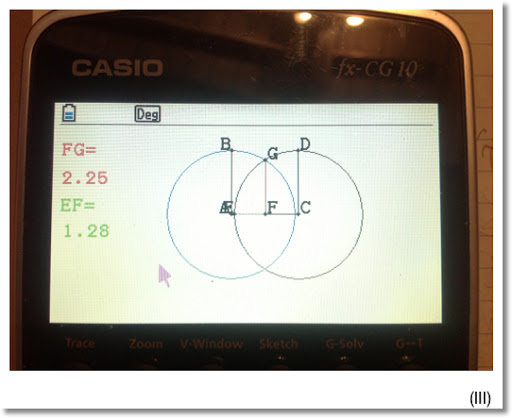At stage (III) ("20 clicks in"), EF = 1.28 and FG = 2.25. Hence the area of the figure in (III) is:

A = 2 * π * 2.64^2 - π * 1.28 * 2.25 ≈ 34.74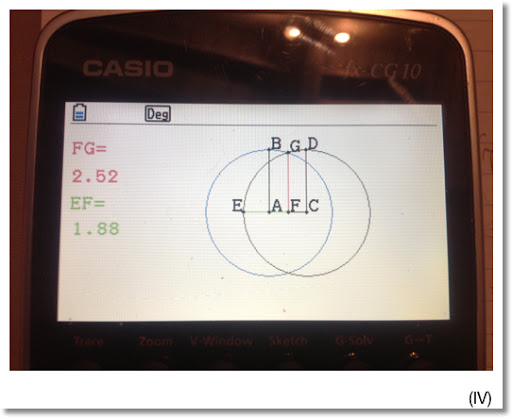At stage (IV) (30 "clicks" in), we have EF = 1.88 and FG = 2.52. Then the area is:

A = 2 * π * 2.64^2 - π * 1.88 * 2.52 ≈ 28.91.

Eventually, the circle outlined in blue meets the circle outlined in black, see (V).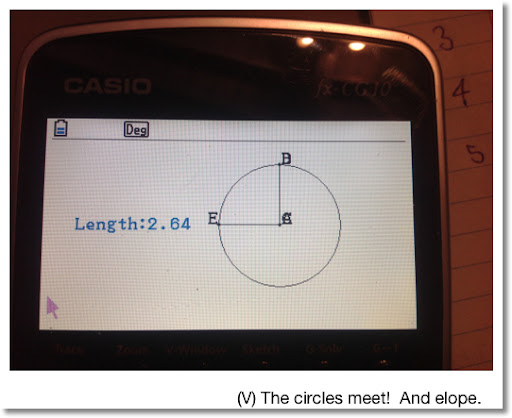The area enclosed by the shape is now A = π * 2.64^2 ≈ 21.90.

I am really enjoying the Casio Prizm. After some use and getting used to the menu structure of the calculator (this applies for most Casio graphing calculators), I find the calculator is easy to use, and I can get to where I want quickly.

Until next time, wishing you good health,

Eddie

This blog is property of Edward Shore. 2013

## Monday, January 7, 2013

### Growing a Circle at an Accelerated Rate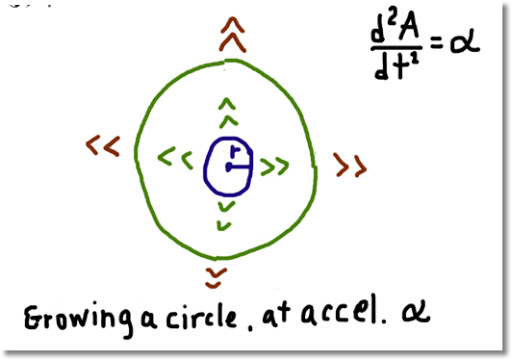Consider a circle. The circle starts as a dot and grows in size by α square units (per time unit). Can we determine when the size of a circle grows to a certain point?

It turns out, we can.

Let A(t) describe the area of the circle at time t. The instantaneous change of the area can be determined by taking the derivative, or A'(t).

The speed of the circle's growth is changing. We determine the speed of the growth by taking the second derivative, A''(t).

In the beginning, the circle starts out as an dot with virtually no area, but has an accelerated growth of α.

(I) A''(t) = α
with initial conditions A(0) = 0 and A'(0) = 0.

Taking the integral of (I):

∫ A''(t) dt = ∫ α dt

A'(t) = α * t + C

With A'(0) = 0,

0 = α * 0 + C,

and C = 0. Hence:

(II) A'(t) = α * t

Applying the integral again, and taking the initial conditions into account:

(III) A(t) = 1/2 * α * t^2

Knowing that the area of a circle with radius r is A = π * r^2; we can relate the circle's radius to the circle's growth by:

(IV) π * r^2 = 1/2 * α * t^2

Examples
1. If a circle grows an accelerated rate of α = 0.1 in^2/s^2, how long will it take for the circle's area to grow to 10 in^2?

With α = 0.1 and A = 10:

10 = 1/2 * (0.1) * t^2

t^2 = √200

t ≈ 14.14214

It takes just over 14 seconds for the circle to grow to 10 square inches.

2. If a circle, from a virtual dot, takes 10 minutes to grow a size where it has radius of 25 inches, determine the its accelerated growth.

The area of a circle at 10 minutes is A(10) = π * 25^2 ≈ 1963.49541 in^2. Then:

A(10) = 1/2 * 10^2 * α

π * 25^2 = 50 * α

α ≈ 39.26991

The acceleration of the circle's growth is about 39.27 in^2/min^2.

Until next time,

Eddie

This blog is property of Edward Shore. 2013

## Saturday, January 5, 2013

### Growing Squares and Circles inside Squares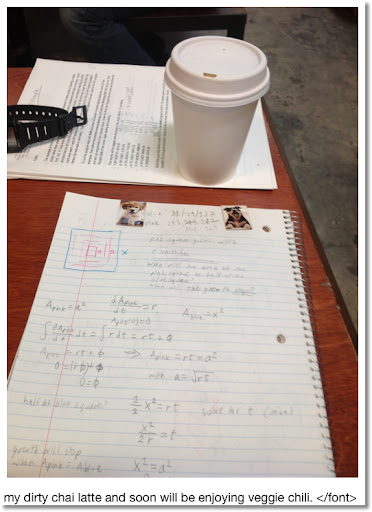Happy New Year everyone! I am blogging from Stories Books and Cafè in Los Angeles. Today's blog is about geometry mixed a little calculus. Let's have fun!

Growing a Square inside a Square

Imagine a blue square with length x. Then from the center of the blue square, a pink square grows at a constant rate r. How long will it take for the pink square to grow as big as the blue square?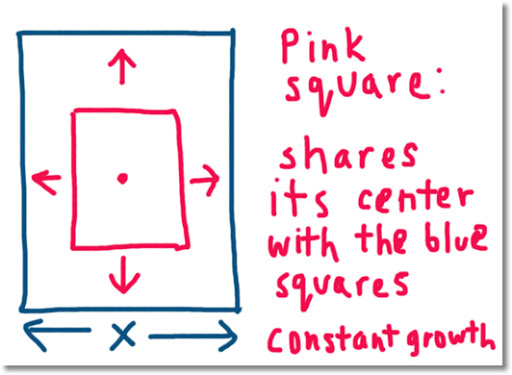Let a = the length of pink's square side. If A represents the area of a square, then let:

Ab = area of the blue square = x² and
Ap = area of the pink square = a².

Note Ap is also a function of time, t, as Ap is changing.

The growth of the pink square can be described as:

dAp/dt = r with the initial condition Ap(0) = 0. Here I assume that the pink square starts out so small it's initial area is negligible.

Then:

∫ dAp/dt dt = ∫ r dt
Ap(t) = r * t + C, for constant C.

Considering the initial condition Ap(0) = 0, implies that C = 0, and Ap(t) = r * t.

So how long does it take for the pink square to grow as big as the blue square? Simply, this happens when the areas of both blue and pink squares equal.

Ab = Ap
x² = r * t
⇒ t = x²/r

For example, if the length of the blue square is x = 5 inches and the rate of the pink square grows at r = 0.5 in/sec, then it takes 5²/0.5 = 50 seconds for the pink square to grow as big as the pink square.

Two Squares Growing

Now let's consider two squares that start out as dots (with virtually no initial area of their own). Start with our blue square with length x and area x^2.

Then place one square, let it be purple and label it A with its center located at (x/4, x/2). Place another square, let it be pink and label it B with its center located at (3x/4, x/2).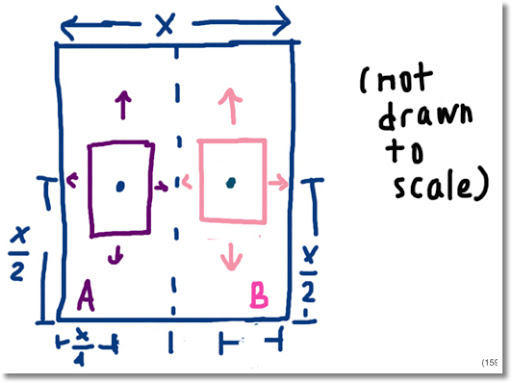Assume the following:

1. The growth of both the purple and pink square is constant, r.

2. The purple and pink squares stop growing when they touch each other.

3. The growth of both squares start simultaneously.

Let:
S = Area of the blue square = x²
A = Area of the purple square
B = Area of the pink square

Both A and B are functions of time, t since they change. Then:

dA/dt = r
∫ dA/dt dt = ∫ r dt
A = r * t + C

Considering the initial condition A(0) = 0, A = r * t.

Likewise, B = r * t.

When do the purple and pink squares meet? Inferring from the picture above, both squares meet when the length of each square reaches the length x/2. Since both squares grow at the same time, we can find out the time for each square by considering only one of the two squares. Without loss of generality, consider the purple square.

The maximum area of A is x²/4. Since A = r * t,

x²/4 = r * t
⇒ t = x²/(4*r)

How big will the two squares get? Considering the maximum size of the purple (and pink) square is x²/4, the largest the two squares will grow (without intersection) is:

x²/4 + x²/4 = x²/2

Growth of a Circle inside of a Square at a Constant Rate

Now consider a circle with radius r with its center located at the center of a square (x/2, x/2). Consider the growth of the circle constant, at rate α. The circle starts as a dot with virtually no area.

How long will it take until the circle grows as big as it can inside the square?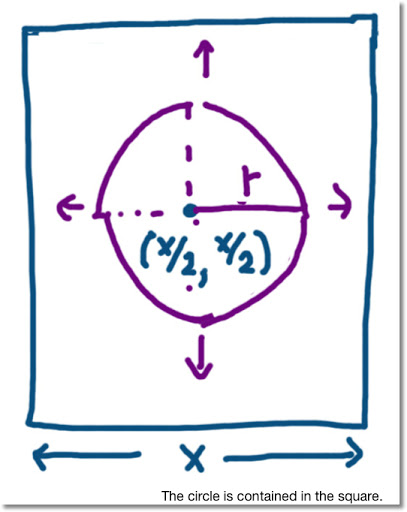The circle will touch the square when its radius extends from the center of the square to one of its edges. But where?

Consider the measure of the radius as a line of coming from the center of the square and growing in a direction parallel to any on of its sides ("up", "down", "left", or "right"). (Hopefully this makes sense). For the moment, allow the circle to grow only when it's entire shape lies inside the square. Then the circle gets to its largest shape when r = x/2.

When will that happen?

Let A be the area of the circle, so A = π * r² and dA/dt = α.

With the initial condition A(0) = 0, A = α * t.

With r = x/2,

α * t = (x/2)² * π
α * t = (x² * π)/4
⇒ t = (x² * π)/(4 * α)

How big is the largest circle? At the largest radius, A = (x² * π)/4

The area of the square is x². Clearly, the area of the square is larger than the circle. Taking a ratio of the circle's area to the square's area:

((x² * π)/4) / x² = π /4 ≈ 0.78540

The largest circle that can be completely contained inside the square has an area that is about 78.5% of the square's area. Cool huh?

What if the Circle is allowed to grow beyond the Square's Borders?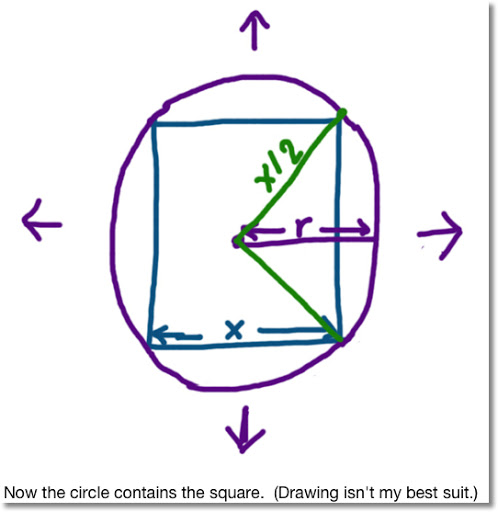At what point does the circle completely encompass the square? In other words, at what point does the circle start containing the square?

This happens with the square's corners, and only its corners touches the circle. Let's determine the area of the circle at this point.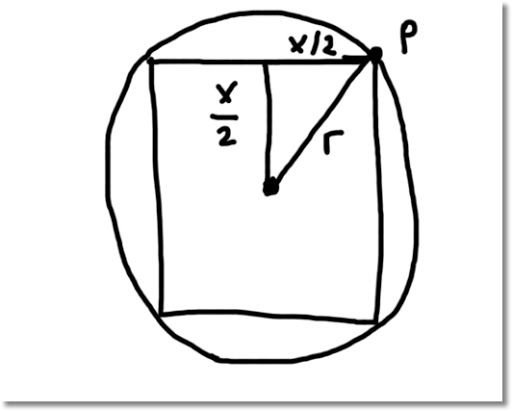Consider the point P in the above diagram. P is also on one of the square's corners. Since the center of the circle and the center of the square are at the same location, the length from point P to the center is r.

Then by the Pythagorean Theorem:

r^2 = (x/2)^2 + (x/2)^2
⇒ r = x/√2

Then the circle's area is A = x^2 * π * 1/2 and the time it takes for the circle to get that large is t = (x^2 * π)/(2 * α).

At this point the area of the circle is π/2, or about 1.57080 times the area of the square.

By the way, the chili was spicy, and good. Thanks and stay safe! Eddie

This blog is property of Edward Shore. 2013

## Tuesday, January 1, 2013

### HAPPY NEW YEAR

HAPPY NEW YEAR!

Have a great 2013!

Many wishes of cheer and happiness!

Time to Celebrate! Be safe!

Eddie

### HP 15C: Error Function and Lower Tail Normal Cumulative Function

HP 15C:   Error Function and Lower Tail Normal Cumulative Function Formulas Used  Error Function  erf(x) = 2 ÷ √π * ∫( e^(-t^2) dt, t = 0 t...